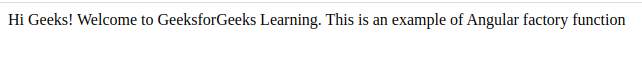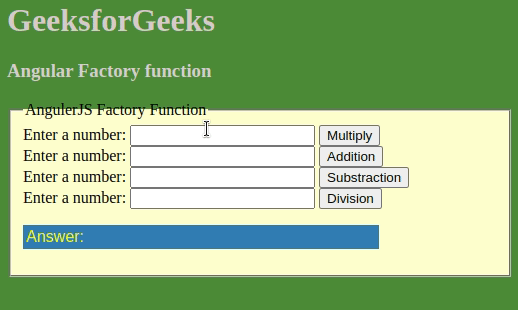GFG App
Open AppBrowser
Continue

# What is the factory function in Angular ?

In Angular, the factory function is always inclined towards class and constructors. Generally, the factory function is used to provide service as a dependency in any angular application. A factory function generates an object, provides it with some logic, executes the function, and returns the object. It is also used to create directives, also used to invoke a method. Most of the time it is used in arithmetic and mathematical operations. Using a factory function provides ease in reusing and maintaining the code of an application. Factory functions are, by default, used to create single-page applications. Since they are used to create single-page applications, therefore their object can be used in multiple instances. They have the freedom to create an object of any type on their own requirement. They are used to create private and protected codes. An interesting fact is a service and factory function generate the same output, but the difference is when which Angular Service should be used.

Syntax:

```var module = angular.module('myapp', []);
module.factory('serviceName', function(){
return serviceObject;
});```

A module is created and a factory function is assigned with a service and some function is linked with it and then the object used in the service is returned after the process. There are many reasons for which  the factory function should be used, among which a few of them are described below:

• If a value is to be returned,  then the factory function should be used.
• By default, Angular uses it in case of service.
• While building an application, if the application is complex then use the factory function.
• If a function is to be used along with mathematical operations or some other function then the factory function should be used.
• When any object is required to be initiated, then the factory function should be used.
• It provides ease while creating private components.
• It primarily provides more flexibility with Javascript functions.

Example 1: In this example, a factory function is created named “myFactory”, in which logic is combined to print a message on the screen. After the logic is executed then the msg i.e. the object that is created is returned after the process is completed.

## HTML

 `` `<``html``>`   `<``head``>` `    ``<``title``>` `        ``Angular Factory Service Example` `    ``` `    ``<``script` `src``=` `"http://ajax.googleapis.com/ajax/libs/angularjs/1.4.8/angular.min.js"``>` `    ``` `    `  `    ``<``script` `type``=``"text/javascript"``>` `        ``var app = angular.module('myApp', []);` `        `  `        ``// Creating Factory` `        ``app.factory('myFactory', function () {` `          ``var displayFact;` `          ``var addMSG = function (msg) {` `            ``displayFact = ' Hi Geeks! Welcome to ' + msg;` `          ``}` `          ``return {` `            ``setMSG: function (msg) {` `              ``addMSG(msg);` `            ``},` `            ``getMSG: function () {` `              ``return displayFact;` `            ``}` `          ``};` `        ``});` `        ``app.controller("myCtrl", function (\$scope, myFactory) {` `            `  `          ``//Inject factory  to controller.` `          ``myFactory.setMSG(` `"GeeksforGeeks Learning. This is an example of Angular factory function");` `          ``\$scope.myCollections = [` `            ``myFactory.getMSG(),` `          ``];` `        ``});` `    ``` ``   `<``body``>` `    ``<``div` `ng-app``=``"myApp"` `ng-controller``=``"myCtrl"``>` `        ``<``div` `ng-repeat``=``"coll in myCollections"``>` `            ``{{coll}}` `        ``` `    ``` ``   ``

Output:Example 2: In this example, the factory function is assigned with the arithmetic operation, which is named “calculatorService”.After all the processes are executed, then the object is returned in the form of output.

## HTML

 `` `<``html``>`   `<``head``>` `    ``<``title``>Angular Factory function` `    ``<``script` `src``=` `"https://ajax.googleapis.com/ajax/libs/angularjs/1.2.13/angular.js"``>` `    ``` `    `  `    ``<``script``>` `    `  `        ``//Defining Factory    ` `        ``var app = angular.module('app', []);` `        `  `        ``app.factory('calculatorService', function () {` `          ``var calculator = {};` `          ``calculator.multiply = function (a, b) { return a * b };` `          ``calculator.add = function (a, b) { return a + b };` `          ``calculator.subtract = function (a, b) { return a - b };` `          ``calculator.divide = function (a, b) { return a / b };` `          ``return calculator;` `        ``});` `        `  `        ``app.controller('CalculatorController', ` `        ``function (\$scope, calculatorService) {` `          ``\$scope.doMultiply = function () {` `            ``\$scope.answer =` `            ``calculatorService.multiply(\$scope.number, \$scope.number);` `          ``}` `          ``\$scope.doAddition = function () {` `            ``\$scope.answer = ` `            ``calculatorService.add(\$scope.number, \$scope.number);` `          ``}` `          ``\$scope.doSubtraction = function () {` `            ``\$scope.answer = ` `            ``calculatorService.subtract(\$scope.number, \$scope.number);` `          ``}` `          ``\$scope.doDivision = function () {` `            ``\$scope.answer = ` `            ``calculatorService.divide(\$scope.number, \$scope.number);` `          ``}` `        ``});    ` `    ``` ``   `<``body` `style``=``"background-color:    #618235;"``>` `    ``<``h1` `style``=``"color: rgb(214, 205, 205);"``>` `        ``GeeksforGeeks` `    ``` `    ``<``h3` `style``=``"color: rgb(214, 208, 208);"``>` `        ``Angular Factory function` `    ``` `    ``<``fieldset` `style``=``"background-color : #FFFACD;"``>` `        ``<``legend``>AngularJS Factory Function` `        ``<``div` `ng-app``=``"app"``>` `            ``<``div` `ng-controller``=``"CalculatorController"``>` `                ``Enter a number:` `                ``<``input` `ng-model``=``"number"` `                       ``type``=``"number"``>` `                ``<``button` `ng-click``=``"doMultiply()"``>` `                    ``Multiply` `                ``<``br``>`   `                ``Enter a number:` `                ``<``input` `ng-model``=``"number"` `                       ``type``=``"number"``>` `                ``<``button` `ng-click``=``"doAddition()"``>` `                    ``Addition` `                `` <``br``>`   `                ``Enter a number:` `                ``<``input` `ng-model``=``"number"` `                       ``type``=``"number"``>` `                ``<``button` `ng-click``=``"doSubtraction()"``>` `                    ``Subtraction` `                `` <``br``>`   `                ``Enter a number:` `                ``<``input` `ng-model``=``"number"` `                       ``type``=``"number"``>` `                ``<``button` `ng-click``=``"doDivision()"``>` `                    ``Division` `                ```   `                ``<``p` `style="font-family:Arial;` `                          ``color:yellow;` `                          ``background:steelblue;` `                          ``padding:3px;` `                          ``width:350px;">` `                    ``Answer: {{answer}}` `                ``` `            ``` `        ``` `    ``` ``   ``

Output:My Personal Notes arrow_drop_up